### TAN Function Examples – Excel, VBA, & Google Sheets

This Tutorial demonstrates how to use the Excel TAN Function in Excel to calculate the tangent.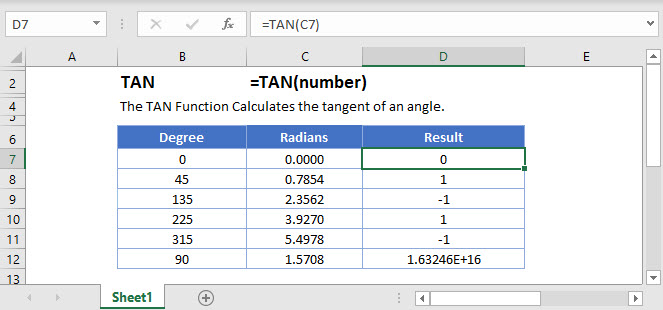## TAN Function Overview

The TAN Function Calculates the tangent of an angle.

To use the TAN Excel Worksheet Function, select a cell and type: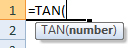(Notice how the formula inputs appear)

## TAN Function Syntax and Inputs:

number – A number.

AutoMacro - VBA Code Generator

## TAN Function

The TAN Function returns the tangent of an angle entered in radians.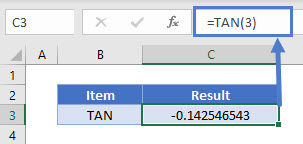## TAN & PI Function

The TAN Function can also return tangent of an angle in degrees if the angle is converted to radians first.

To convert the angle in degrees to radians we can use the PI Function.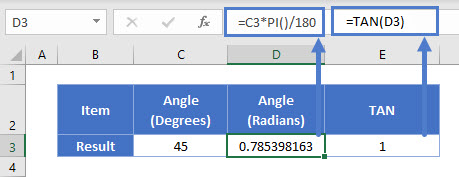## TAN & DEGREES Function

Alternatively, the RADIANS Function can be directly used to convert angle in degrees to radians.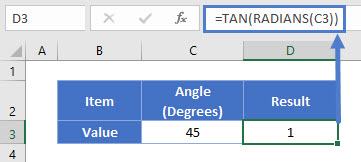## TAN Function – Negative Angle

The TAN Function can also return tangent of a negative angle.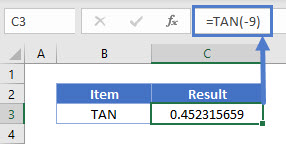## TAN Function in Google Sheets

The TAN Function works exactly the same in Google Sheets as in Excel: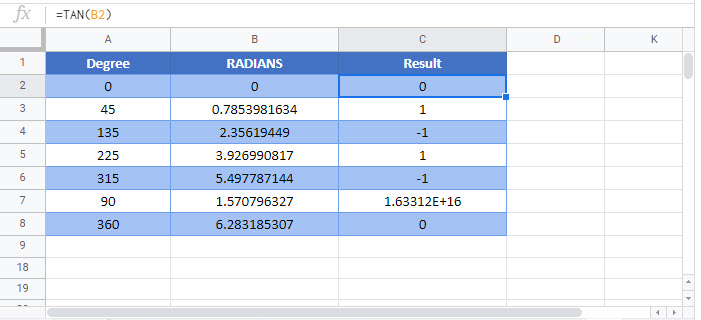## Additional Notes

Use the TAN Function to calculate the tangent of an angle. Simply enter the angle directly into the function or reference a cell containing the angle.

## TAN Examples in VBA

You can also use the TAN function in VBA. Type:
`application.worksheetfunction.tan(number)`
For the function arguments (number, etc.), you can either enter them directly into the function, or define variables to use instead.

Return to the List of all Functions in Excel

## Excel Practice Worksheet

Practice Excel functions and formulas with our 100% free practice worksheets!

• Automatically Graded Exercises
• Learn Excel, Inside Excel!